Courses

# Test: Exponents And Powers - 1

## 20 Questions MCQ Test Mathematics (Maths) Class 7 | Test: Exponents And Powers - 1

Description
This mock test of Test: Exponents And Powers - 1 for Class 7 helps you for every Class 7 entrance exam. This contains 20 Multiple Choice Questions for Class 7 Test: Exponents And Powers - 1 (mcq) to study with solutions a complete question bank. The solved questions answers in this Test: Exponents And Powers - 1 quiz give you a good mix of easy questions and tough questions. Class 7 students definitely take this Test: Exponents And Powers - 1 exercise for a better result in the exam. You can find other Test: Exponents And Powers - 1 extra questions, long questions & short questions for Class 7 on EduRev as well by searching above.
QUESTION: 1

### Express 256 as a power 4.

Solution:

4*4*4*4 = 256

256 = 44

So option B is the correct answer.

QUESTION: 2

### Express 729 as a power of 3.

Solution: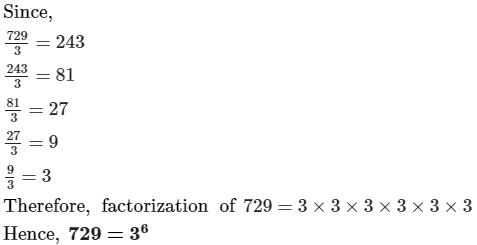So option C is the correct answer.

QUESTION: 3

### Express 2048 as a power 2.

Solution:

2048 = 2 x 1024

= 2 x 2 x 512

= 2 x 2 x 2 x 256

= 2 x 2 x 2 x 2x 128

= 2 x 2 x 2 x 2 x 2 x 64

= 2 x 2 x 2 x 2 x 2 x 2 x 32

= 2 x 2 x 2 x 2 x 2 x 2 x 2 x 16

= 2 x 2 x 2 x 2 x 2 x 2 x 2 x 2 x 8

= 2 x 2 x 2 x 2 x 2 x 2 x 2 x 2 x 2 x 2 x 2

= 211

So option B is the correct answer.

QUESTION: 4

Which of the following is the value of (4 / 5)-9 / (4 / 5)9?

Solution: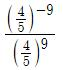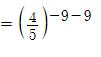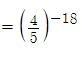QUESTION: 5

Express 432 as a product of powers of prime factors.

Solution:

Prime factorization of 432 is,

432 =2×2×2×2×3×3×3

to express this as power

432=(2)×(3)³

QUESTION: 6

The value of (−1)55 is

Solution:

If any power having a odd number is raised to -1 then result will be -1

So (−1)55 = -1

So option B is correct answer.

QUESTION: 7

The value of (−1)500 is

Solution:

If any power having a even number is raised to -1 then the  result will be 1

Since the power is an even number , So (−1)500 =  1

QUESTION: 8

The value of (−1)47 is

Solution:

If any power having a odd number is raised to -1 then result will be -1

So (−1)47 = -1

So option A is correct answer.

QUESTION: 9

The value of (−1)400 is

Solution:

If any power having an even number is raised to -1 then the  result will be 1

Since the power is an even number , So (−1)400 =  1

So option A is the correct answer.

QUESTION: 10

Anu wanted to score 26 points in the game of cards, but ends up only 8/3 points. By how many points did she fail short?

Solution:

Points by which Anu fell short is

= 26 - 8/3 = (78 - 8)/3

= 70/3

QUESTION: 11

Simplify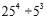Solution:

254/5= (52)4/53=58/53=58-3=55

QUESTION: 12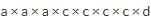can de written in exponential form as

Solution:

a × a × a × c × c × c × c × d = a3 × c4 × d

QUESTION: 13

512 can de written in exponential form as

Solution:

512=8*8*8=83=(23)3=23*3=29

QUESTION: 14

What is the reciprocal of (-3 / 4)0?

Solution:

Anything raised to the power zero is 1 .

QUESTION: 15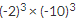can be written as

Solution:

(-2)3(-10)3=(-2)*(-2)*(-2)*(-10)*(-10)*(-10), which is the expansion of the exponential forms which means the power depicts how many times a number is multiplied.

QUESTION: 16

In 2the exponent is

Solution:

Exponents, or powers, are a way of indicating that a quantity is to be multiplied by itself some number of times. In the expression 252 is called the base and 5 is called the exponent, or power.

QUESTION: 17

The value of (4- 20) x 5is

Solution:

Anything raised to the power zero is 1 .

In the given expression,  (4- 20) x 50

= (1 - 1) x 1

= ( 0 ) x 1

= 0

So Option B is correct answer.

QUESTION: 18

If a = 3 and b = 4 then find the value of aa + bb

Solution:

Given, a = 3 and b = 4

aa + b= 33 + 4= 3 x 3 x 3 + 4 x 4 x 4 x 4

= 27 + 16 x 16

= 27 + 256

= 283

So option A is the correct answer.

QUESTION: 19

(2/7)2 x (2/7)4 is equal to:

Solution:

Whenever you multiply two terms with the same base, you can add the exponents:

( x m ) ( x n ) = x( m + n )

So (2/7)2 x (2/7)4  = (2/7)6

So option C is the correct answer.

QUESTION: 20

1 lakh is equal to

Solution:

1 lakh is equal to 10

So option A is the correct answer.